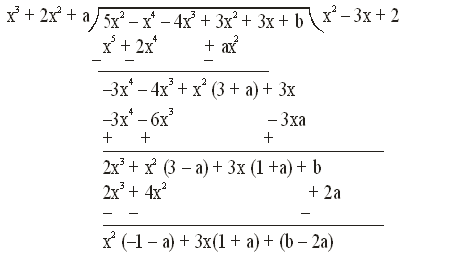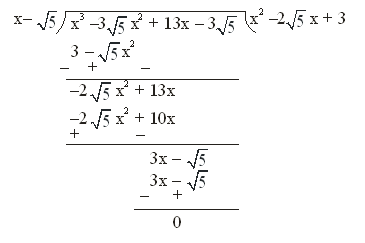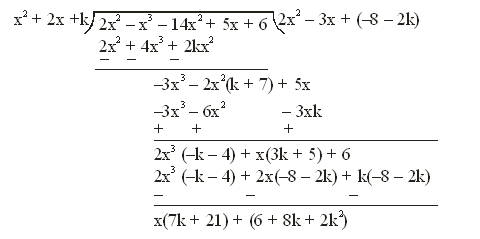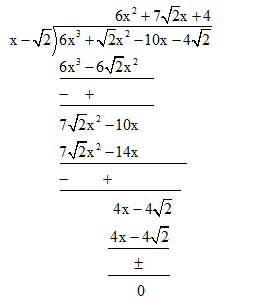Filter By

## All Questions

#### For which values of a and b, are the zeroes of  also the zeroes of the polynomial ? Which zeroes of p(x) are not the zeroes of q(x)?

Solution.

Given :-

,

Hence, q(x) is a factor of p(x) use divided algorithmSince is a factor hence remainder = 0

By comparing

– 1 – a = 0                               b – 2a = 0

a = – 1                                     2a = b

put a = –1

2(–1) = b

b = –2

Hence, a = –1, b = –2

x – 2 = 0                                  x – 1 = 0

x = 2                                        x = 1

Hence, 2, 1 are the zeroes of p(x) which are not the zeroes of q(x).

#### Given that  is a factor of the cubic polynomial ,find all the zeroes of the polynomial.

Solution.

The given cubic polynomial is

Hence  is a factor

Use divided algorithma = 1, b =  , c = 3

Hence,   are the zeroes of the polynomial.

## Crack CUET with india's "Best Teachers"

• HD Video Lectures
• Unlimited Mock Tests
• Faculty Support#### Find k so that is a factor of . Also find all the zeroes of the two polynomials.

The given polynomial is

Here, is a factor of

Use division algorithmHere, is remainder

It is given that is a factor hence remainder = 0

By comparing L.H.S. and R.H.S.

7k + 21 = 0

k = – 3                         ….(1)

2k(k + 1) + 6(k + 1) = 0

(k + 1) (2k + 6) = 0

k = –1, –3                    …..(2)

From (1) and (2)

k = –3

Hence,

Put k = –3

x + 3 = 0                      x – 1 = 0                      x – 2 = 0                      2x + 1 = 0

x = –3                          x = 1                            x = 2                            2x = – 1

Here zeroes of  is –3, 1

Zeroes of

#### Given that is a zero of the cubic polynomial , find its other two zeroes.

Given cubic polynomial is

If  is a zero of the polynomial then (x – ) is a factor of=0

Hence,  are the other two zeroes.

## Crack NEET with "AI Coach"

• HD Video Lectures
• Unlimited Mock Tests
• Faculty Support#### Given that the zeroes of the cubic polynomial are of the form a, a + b, a + 2b for some real numbers a and b, find the values of a and b as well as the zeroes of the given polynomial.

Solution. Here the given cubic polynomial is

A = 1, B = –6, C = 3, D = 10

Given that,

We know that,

3(a + b) = 6

a + b = 2                                  …..(1)

(Q  a + b = 2)

(Q  a + b = 2)

a = 5, –1

Put a = 5 in (1)                                    put a = –1 in (1)

5 + b = 2                                              –1 + b = 2

b = –3                                                  b = 3

Hence, value of a = 5, b = –3 and a = –1, b = 3

put a = 5, b = –3 and we get zeroes

a = 5

a + b = 5 – 3 = 2

a + 2b = 5 + 2(–3) = –1

Hence, zeroes are 5, 2, –1.

#### For each of the following, find a quadratic polynomial whose sum and product respectively of the zeroes are as given. Also find the zeroes of these polynomials by factorisation.(i) (ii) (iii) (iv)

Solution. Zeroes : zeroes of the polynomial are the value(s) that makes it equal to 0.

Here, sum of zeroes

Product of zeroes

Let p(x) is the required polynomial

p(x) = x2 – (sum of zeroes)x + (product of zeroes)

Multiply by 3

Hence, is the required polynomial

=0

are the zeroes of p(x).

Solution. Zeroes : zeroes of the polynomial are the value(s) that makes it equal to 0.

Here, sum of zeroes

Product of zeroes

Let p(x) is the required polynomial

p(x) = x2 – (sum of zeroes)x + (product of zeroes)

Multiply by 16 we get

Hence, is the required polynomial

=

==0

are the zeroes of p(x).

Solution. Zeroes : zeroes of the polynomial are the value(s) that makes it equal to 0.

Here, sum of zeroes

Product of zeroes = –9

Let p(x) is required polynomial

p(x) = x2 – (sum of zeroes)x + (product of zeroes)

Hence,  is the required polynomial

=

=

==0

are the zeroes of p(x)

Solution. Zeroes : zeroes of the polynomial are the value(s) that makes it equal to 0.

Here sum of zeroes

Product of zeroes

Let p(x) is the required polynomial

p(x) = x2 – (sum of zeroes)x + (product of zeros)

Multiplying by  we get

Hence,  is the required polynomial

=

=

are the zeroes of p(x).

## Crack JEE Main with "AI Coach"

• HD Video Lectures
• Unlimited Mock Tests
• Faculty Support#### prove that:

prove that:

Taking left hand side

Now using the identity

So,

Now using

So equation (i) becomes:

Now,

Hence proved.

#### If a + b + c = 5 and ab + bc + ca = 10, then prove that a3 + b3 + c3 –3abc = – 25

Given: (a + b + c) = 5, ab + bc + ca = 10

To Prove: a3 + b3 + c3 –3abc = – 25

We know that

Now,

Putting the values, we get:

Hence proved.

## Crack CUET with india's "Best Teachers"

• HD Video Lectures
• Unlimited Mock Tests
• Faculty Support#### If a, b, c are all non-zero and a + b + c = 0, prove that

Given,

L.H.S.

Taking LCM of denominators, we get

L.H.S

Now we know that if a + b + c = 0 then

Putting the value in above equation:

L.H.S = = 3 = RHS

Hence proved.

#### Multiply: by

Solution

We have,

This can be written as:

We know that

So comparing the RHS of equation (i) with the above identity:

a = x

b = -2y

c = -z

We get: